# CBSE Class 12 Economics Consumers Equilibrium and Demand Revision Notes

POINTS TO REMEMBER:-

Consumer: is an economic agent who consumes final goods and services to satisfy wants.

Utility:- It is the want satisfying power of a commodity. This is measured in terms of ‘utils’-

Utility can be of two types.

(a)     Total Utility:- TU is the sum total of satisfaction  that the consumer derives when a certain number of units of a particular commodity are consumed.

Tux= f(Qx) or TU = ∑ MU

(b)     Marginal Utility:- It is a change in total utility by consuming an additional unit of a commodity or in other words MU is the additional satisfaction derived from the consumption of an additional unit of the commodity.

MUn = TUn – TUn-1

Alternatively: MUx= ∆ TUx/ ∆/Qx

Law of Diminishing Marginal Utility : As consumer consumes more and more units of commodity, the Marginal utility derived from the  each successive unit goes on declining.

Consumer Equilibrium :-Consumer equilibrium refers to  a situation when a consumer maximizes her satisfaction out of her given money income and the price of the desired good(s) and there is no tendency to deviate from that situation.

Conditions of consumer equilibrium

In case of one good:

MUx/PX=Mum   or MUx / MUm=PX or Mux=PX

Let the consumer consume a good X and its price is  and MU derived from it is .

The condition of consumer equilibrium will be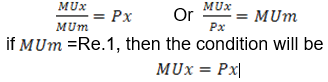ORDINAL UTILITY ANALYSIS (IC ANALYSIS)

Introduction:

Two English economists J. R. Hicks and R.G.D. Allen fired heavily shells at cardinal utility theory and propounded indifference curve approach based upon the notion of ordinal utility. It implies that utility being psychological concepts cannot be measured cardinally rather they can be ranked. Indifference Curve method was evolved to supersede the cardinal utility analysis of demand.

Budget set/ Budget Space:

Budget set is the collection of all those consumption bundles which costs the consumer exactly equal or strictly less than two her money income. Mathematically:

P1x1+P2x2 ≤  M

Budget Line/ Price Line:

It is the locus of points of all those consumption bundles which costs the consumer exactly equal to her money income. Mathematically:

P1x1+P2x2 = M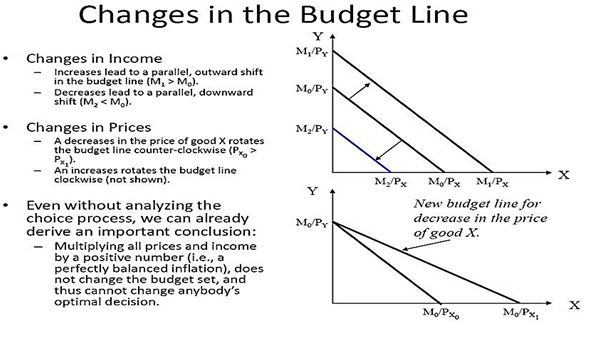(i)          Monotonic preferences:

A consumer’s preference is said to be monotonic if and only if the preferred bundle consists of more of at least one good and no less of the other good in comparison to the non-preferred bundle.

INDIFFERENCE CURVE (IC) :

Indifference Curve (IC) is the locus of points of all those consumption bundles among which the consumer’s preference is indifferent. In other wards it is the locus of points of all the consumption bundles which gives the consumer equal level of satisfaction.

Indifference Map:

It is the family (Collection) of indifference curvesMarginal Rate of Substitution (MRS):

MRS is the rate of sacrifice of one good in order to consume an additional unit of another good along an Indifference curve. This can be under stood from the following table and diagram.

It is measured byThis MRS xy is nothing but the slope of the Indifference Curve.

Properties of Indifference Curve:

(a) Indifference curve is downward sloping from left to right

(b) Indifference curve is convex to the point of origin

(c) Higher IC represents higher level of satisfaction

(d) Two IC’s can never intersect each other

Consumer’s equilibrium by IC Analysis (Hicks and Allen approach) Conditions:

• First order/Necessary Conditions: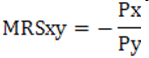Or,   Slope of IC = Slope of Budget Line

Or, Budget Line is tangent to the IC

• Second Order / Sufficient Conditions:

At the point of tangency IC must be convex to the point of origin Or , MRSxy must be diminishing

DEMAND

Introduction:

We are interchangeably using the word desire , want and demand. In a layman’s point of view demand means effective desire to have a commodity backed by willingness to pay and ability to pay.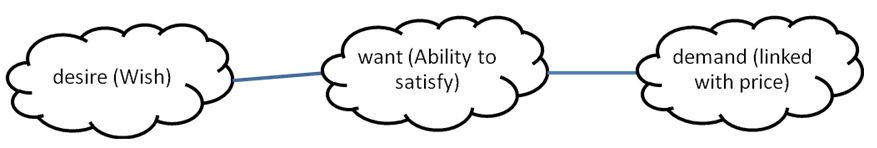In economics demand is something different. The demand for a commodity is essentially consumer’s attitude and reaction towards that commodity. This consumer’s attitude gives rise to actions in purchasing a certain number of units of a commodity at various given prices.

Meaning:

Demand refers to various quantities of a particular commodity that a consumer is ready to buy at different prices.

Types of demand:

Demand is two types:

• Individual demand
• Market demand

Individual demand -It refers to various quantities of a particular commodity that a particular consumer is ready to buy at its various possible prices

Determinants

Followings are the determinants of individual demand

• Own price: when price of commodity is fall its quantity demand increases and when its price rises its quantity demand decrease

• Price of related goods: the quantity demand of a commodity is affected by the price of its related commodity. Like its substitutes and complementary goods

• Substitute Goods: Two goods are called substitute to each other when price of one good and quantity demand of the other varies directly with each other. In simple language those goods which are used one in others place are called substitute typo each other. Ex: Tea and Coffee

• Complementary Goods: Two goods are called complementary to each other when price of one good and quantity demand of the other varies inversely with each other. In simple language those goods which are used jointly are called substitute typo each other. Ex: Tea and Sugar

• Income of the consumer: When income of a consumer rises its quantity demand will increase and when income falls its quantity demand decreases

• Tastes and preferences: if a commodity is in fashion or preferred by the consumer then its demand will increase and if it is out of fashion its demand will decrease.

Demand schedule:

Tabular representation of various quantities of a particular commodity that a particular consumer is ready to buy at its various possible prices is called a demand schedule.

Demand curve:

Graphic representation of a demand schedule which represents various quantities of a particular commodity that a particular consumer is ready to buy at its various possible prices is called a demand curveDemand Function:

Functional relationship between quantity demand of a commodity and its various determinants

qx = f(Px,Pr,I,T)

qx = Quantity demand of commodity-X , Px= Price of commodity X , Pr = Price of related commodity of X , I = Income of the consumer , T = Taste of the consumer

but in general we keep other determinants constant and draw the relationship between price of a commodity and its quantity demand

q = f(p)

In a typical linear equation the demand equation is

q = a-bp

Here a is the vertical intercept and (-) b is the slope of the demand curve: (-)(Rate at which q changes with respect to p)

Slope of a demand curve:

♦ Slope of the demand curve measures the rate at which demand changes with respect to its price

♦ Slope of a curve is derived by the ratio of change in the dependent variable to change in the independent variable and through a graph by the ratio of change in the variable in vertical axis to change in the variable in horizontal axis

♦ Generally we take the independent variable in horizontal axis and dependent variable in vertical axis But in case of the demand curve we are taking the reverse

♦ So the demand curve is an inverse demand curve ,Therefore its slope as per the curve is the inverse as per the equationMarket demand:

It refers to various quantities of a particular commodity that all consumers in the market are ready to buy at its various possible prices. It is the summation of individual demands.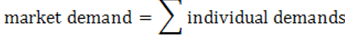Law of demand

Statement:

Other things remaining constant (Ceteris Paribus) when price of a commodity rises its quantity demand decreases and when price of a commodity falls its quantity demand decreases

Assumptions:

• Income of the consumer remain constant

• Price of the substitute and complementary goods remain constant

• Taste and preferences of the consumer remain constant

Change in quantity demanded: (Movement along the demand curve)

When quantity demand of a commodity changes due to change in its own prices and other determinants remaining constant it is called change in demand

This is of two types:

(a) Expansion/extension in demand:

• When quantity demand of a commodity increases due to fall in its price in this case there is upward movement along the demand curve

(b) Contraction in demand:

• When quantity demand of a commodity  increases due to fall in price in this case there is upward movement along the demand curve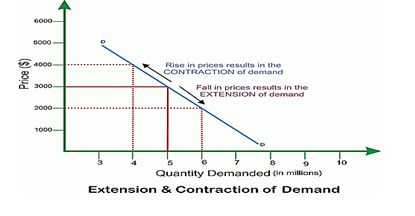Change in demand (Shift in demand curve)

When quantity demand of a commodity changes due to change in its other determinants and own price remaining constant it is called change in demand

Types:

This is of two types:

(i)  Increase  in demand:

♦ When quantity demand of a commodity increases due to favorable change in its other determinants like income , price of related commodity and own price remaining constant

♦ In this case there is right ward parallel shift of the  demand curve

(ii)  Decrease  in demand:

♦ When quantity demand of a commodity decreases due to unfavorable change in its other determinants like income , price of related commodity and own price remaining constant

♦  In this case there is left ward parallel shift of the  demand curveDistinction between change in demand and change in quantity demand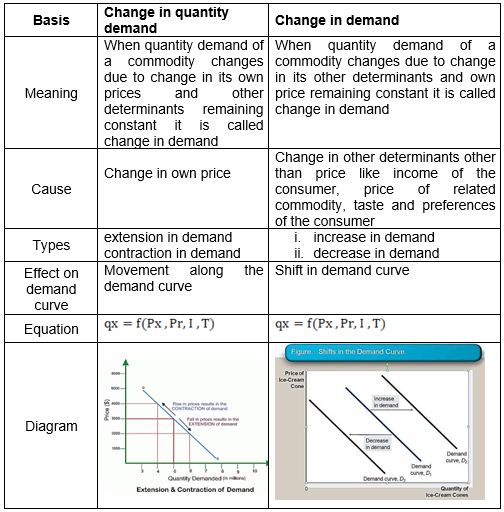Distinction between extension in demand and increase in demand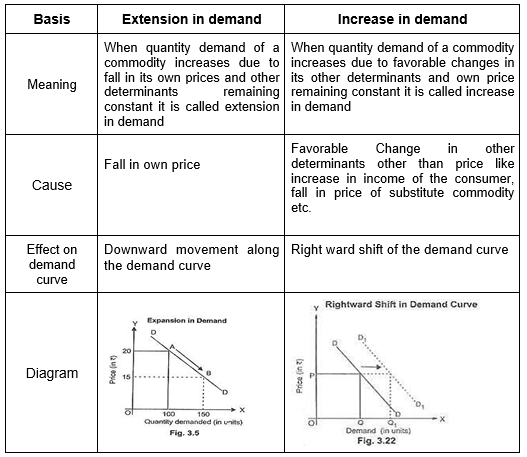Distinction between contraction in demand and decrease in demand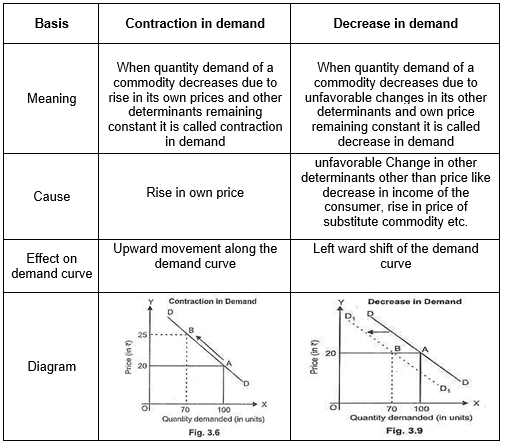Distinction between extension in demand and contraction in demand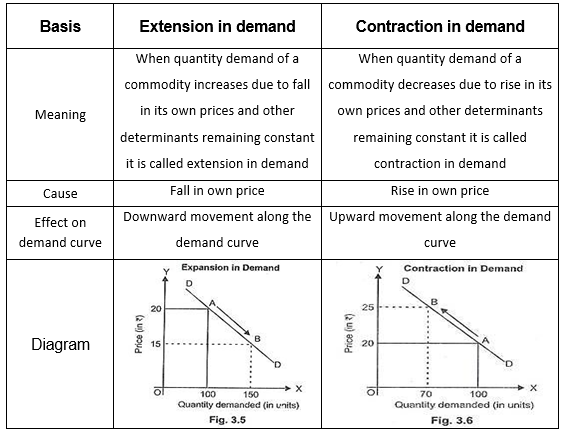Distinction between increase in demand and decrease in demandEffect of change in income of the consumer on quantity demand of a commodity

The effect of change in income of a commodity on quantity demand of a commodity can be explained in case of two types of commodity

Case-I: Normal good:

Meaning:

A good is called normal good when its quantity demand varies directly with change in income of the consumer. It implies that when income of the consumer rises its quantity demand increase and when income of the consumer falls its quantity demand decrease. For example good quality rice

The effect on change income can be explained for the two cases When income increases:

• In this table when the income of the consumer increase from 100 to 200 units its quantity demand increase from 10 to 20 units

• In the diagram the original demand curve is D

• When income increased the demand curve shifts rightward as D1

This implies there is increase in demandWhen income decreases

• In this table when the income of the consumer decrease from 200 to 100 units its quantity demand decrease from 20 to 10 units

• In the diagram the original demand curve is D

• When income decreased the demand curve shifts leftward as D1

• This implies there is decrease in demand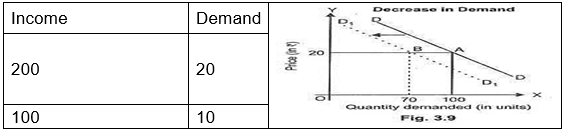Case-II: Inferior good:

Meaning:

A good is called inferior good when its quantity demand varies inversely with change in income of the consumer. It implies that when income of the consumer raises its quantity demand decrease and when income of the consumer falls its quantity demand increase. For example low quality rice

The effect on change income can be explained for the two cases

When income increases:

• In this table when the income of the consumer increase from 100 to 200 units its quantity demand decrease from 20 to 10 units

• In the diagram the original demand curve is D

• When income increased the demand curve shifts leftward as D1

• This implies there is decrease in demandWhen income decreases

• In this table when the income of the consumer decrease from 200 to 100 units its quantity demand increase from 10 to 20 units

• In the diagram the original demand curve is D

• When income decreased the demand curve shifts rightward as D1Effect of change in price of related commodity on quantity demand of a commodity

The effect of change in price of related commodity on quantity demand of a commodity can be explained in case of two types of commodities.

Case-I : Substitute / Competitive goods

Meaning:

In a layman’s point of view two goods are called substitute to each other when they are used one in others place.

But in economic sense two goods are called substitute to each other when price of one good and quantity demand of the other varies directly with each other. It implies when price of one good increases quantity demand of the other increases and when price of one good decreases quantity demand of the other decreases. For example: Tea and Coffee, rice and bread, pen and pencil etc

The effect of change in price of substitute good can be explained under the two cases - case of tea and coffee

When price of substitute good increases

• When price of tea increases , the consumer will consume less amount of tea (due to law of demand)

• At the same time price of coffee remaining constant it will became comparative cheaper to tea

• As coffee is used in place of tea so the consumer will substitute tea for coffee

• As result the quantity demand of coffee will increase

• In this diagram the original demand curve of coffee is D

• Due to rise in price of tea the demand curve of coffee will shift rightward as D1

• This implies there is increase in demand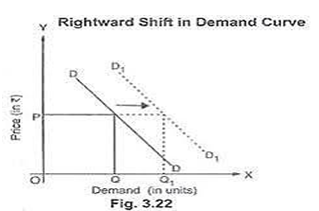When price of substitute good decreases

• When price of tea decreases, the consumer will consume more amount of tea (due to law of demand)

• At the same time price of coffee remaining constant it will became comparative costlier to tea

• As coffee is used in place of tea so the consumer will substitute coffee for tea

• As result the quantity demand of coffee will decrease

• In this diagram the original demand curve of coffee is D

• Due to fall in price of tea the demand curve of coffee will shift leftward as D1

• This implies there is decrease in demandCase-II : Complementary / Supplementary goods

Meaning:

In a layman’s point of view two goods are called complementary to each other when they are jointly demanded.

But in economic sense two goods are called complementary to each other when price of one good and quantity demand of the other varies inversely with each other. It implies when price of one good increases quantity demand of the other decreases and when price of one good decreases quantity demand of the other increases. For example: Tea and Sugar, Shoe and Socks, Car and petrol etc.

The effect of change in price of complementary good can be explained under the two cases – case of tea and sugar

When price of complementary good increases

• When price of tea increases , the consumer will consume less amount of tea (due to law of demand)

• As sugar is jointly demanded with tea the demand for sugar will also decreases

• In this diagram the original demand curve of sugar is D

• Due to rise in price of tea the demand curve of sugar will shift leftward as D1

• This implies there is decrease in demand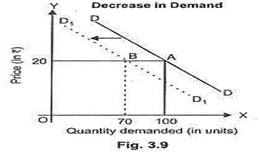When price of complementary good decreases

• When price of tea decreases , the consumer will consume more amount of tea (due to law of demand)

• As sugar is jointly demanded with tea the demand for sugar will also increases

• In this diagram the original demand curve of sugar is DD1

• Due to fall in price of tea the demand curve of sugar will shift rightward as D2D3

• This implies there is increase in demand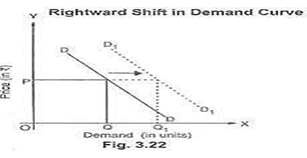ELASTICITY OF DEMAND

The Law of demand which you have studied in the last chapter tells you only the direction of change in price and quantity demand of a commodity. It does not tell you about the intensity of relationship between price and quantity demand of a commodity. It is captured by the concept of “Elasticity of Demand”

PRICE ELASTICITY OF DEMAND

Meaning:

It is the degree of responsiveness of change in quantity demand of a commodity due to change in its price.

Properties:

• It is a pure number i.e. free from the unit of measurement

• It is always express with a minus(-) sign as there is inverse relationship between price and quantity demand of a commodity

• The elasticity of demand associated with a flatter demand curve is more in comparison to a steeper demand curve

Degrees of elasticity of demand:

The extent of responsiveness of demand with change in the price is not always the same.

The demand for a product can be elastic or inelastic, depending on the rate of change in the demand with respect to change in price of a product.

Elastic demand is the one when the response of demand is greater with a small proportionate change in the price. On the other hand, inelastic demand is the one when there is relatively a less change in the demand with a greater change in the price.

For better understanding the concepts of elastic and inelastic demand, the price elasticity of demand has been divided into five types, which are shown in Figure-1:(a)         Perfectly Elastic Demand:

When a small change in price of a product causes a major change in its demand, it is said to be perfectly elastic demand.

In perfectly elastic demand, a small rise in price results in fall in demand to zero, while a small fall in price causes increase in demand to infinity.

In such a case, the demand is perfectly elastic or ep = ∞

The degree of elasticity of demand helps in defining the shape and slope of a demand curve. Therefore, the elasticity of demand can be determined by the slope of the demand curve. Flatter the slope of the demand curve, higher the elasticity of demand.

In perfectly elastic demand, the demand curve is represented as a horizontal straight line, which is shown in the following figure 2.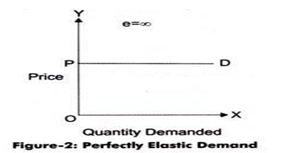• Though, perfectly elastic demand is a theoretical concept and cannot be applied in the real situation. However, it can be applied in cases, such as perfectly competitive market and homogeneity products. In such cases, the demand for a product of an organization is assumed to be perfectly elastic.

### (b)         Perfectly Inelastic Demand:

• A perfectly inelastic demand is one when there is no change produced in the demand of a product with change in its price.

• The numerical value for perfectly inelastic demand is zero (ep=0).

In case of perfectly inelastic demand, demand curve is represented as a straight vertical line, which is shown in Figure-3: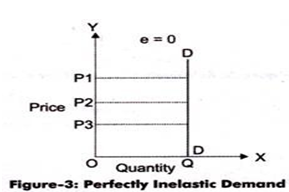Perfectly inelastic demand is a theoretical concept and cannot be applied in a practical situation. However, in case of essential goods, such as salt, the demand does not change with change in price. Therefore, the demand for essential goods is perfectly inelastic.

### • The numerical value of relatively elastic demand ranges between one to infinity. (ep>1)

The demand curve of relatively elastic demand is gradually sloping, as shown in Figure-4:### • The numerical value of relatively elastic demand ranges between zero to one (ep<1). Marshall has termed relatively inelastic demand as elasticity being less than unity.

The demand curve of relatively inelastic demand is rapidly sloping, as shown in Figure-5### • A curve is a rectangular hyperbola when area under the curve is same at every point

Mathematically,  pq = c

The demand curve for unitary elastic demand is represented as a rectangular hyperbola, as shown in Figure-6: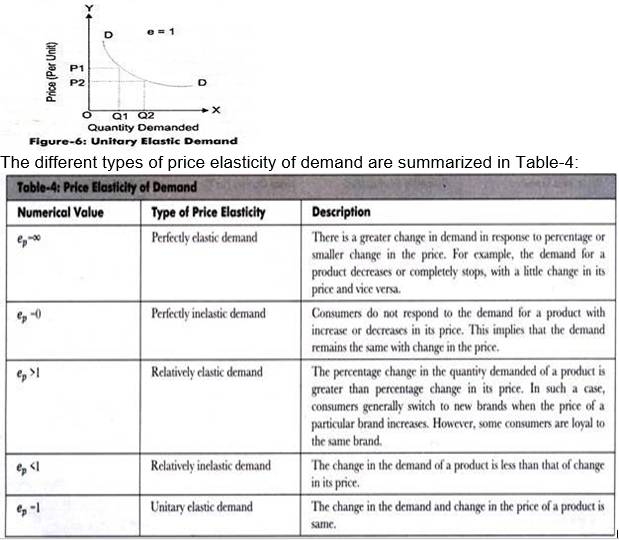Percentage method of measuring elasticity of demand

There are basically three methods of measuring elasticity of demand.

In this method elasticity of demand is measured as a ratio between change in quantity demand to change in price of a commodity

Mathematically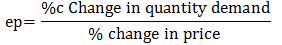## Tags:

Click for more Economics Study Material
 CBSE Class 12 Economics Producer Behaviour and Supply Revision Notes
 CBSE Class 12 Economics 2015 examination guidance CBSE Class 12 Economics Introduction Revision Notes
 CBSE Class 12 Economics Consumers Equilibrium and Demand Revision Notes
 CBSE Class 12 Economics Forms of Market Revision Notes CBSE Class 12 Economics National Income Revision Notes
 CBSE Class 12 Economics Money and Banking Revision Notes
 CBSE Class 12 Economics Determination of Income and Employment Revision Notes CBSE Class 12 Economics Income and Employment Revision Notes
 CBSE Class 12 Economics Balance of Payment Revision Notes

## Latest NCERT & CBSE News

Read the latest news and announcements from NCERT and CBSE below. Important updates relating to your studies which will help you to keep yourself updated with latest happenings in school level education. Keep yourself updated with all latest news and also read articles from teachers which will help you to improve your studies, increase motivation level and promote faster learning

### Surya Namaskar Project on 75th Anniversary of Independence Day

Ministry of Education, Govt of India vide letter No. F.No. 12-5/2020-IS-4 dated 16.12.2021 has intimated that under the banner Azadi ka Amrit Mahotsav the National Yogasanasports Federation has decided to run a project of 750 million Surya Namaskar from 01 January 2022...

### National Youth Day and Birth Anniversary of Swami Vivekananda

Ministry of Education, Govt. of India vide D.O No. 12-4/2021-IS.4 dated 04.01.2022 intimated that 12 January 2022 will be celebrated as “National Youth Day” and “Birth Anniversary of Swami Vivekananda”.   All Schools affiliated to CBSE may celebrate 12 January 2022 as...

### Board Exams Date Sheet Class 10 and Class 12

Datesheet for CBSE Board Exams Class 10  (Scroll down for Class 12 Datesheet) Datesheet for CBSE Board Exams Class 12